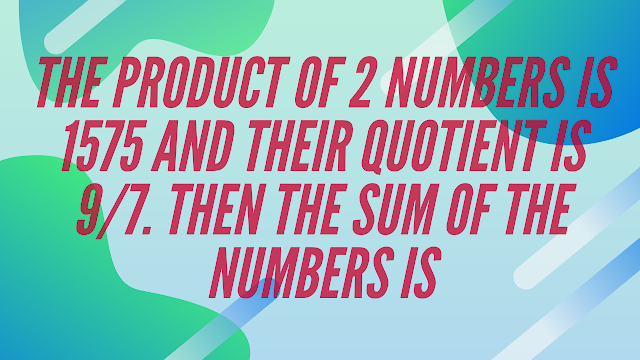Friday, February 3, 2023
HomeGeneral AptitudeThe product of 2 numbers is 1575 and their quotient is $frac{9}{7}$....

# The product of 2 numbers is 1575 and their quotient is $frac{9}{7}$. Then the sum of the numbers is

##Question:

The product of 2 numbers is 1575 and their quotient is $frac{9}{7}$. Then the sum of the numbers is

a.   74

b.   78

c.   80

d.   90

Let the numbers be x and y.
$therefore xy=1575$ and $frac{x}{y}=frac{9}{7}$
$therefore frac{xy}{(frac{x}{y})}=frac{1575}{(frac{9}{7})}$
$therefore y^2=1225$
$therefore y=35$
Hence, $x=45$
So, sum of the numbers
$=x+y$
$=35+45$
$=80$
RELATED ARTICLES

### Dimension theorem of a quotient space: If W be a subspace of a finite dimensional vector space V over ​( mathbb{R} )​ then ​(...

$${}$$